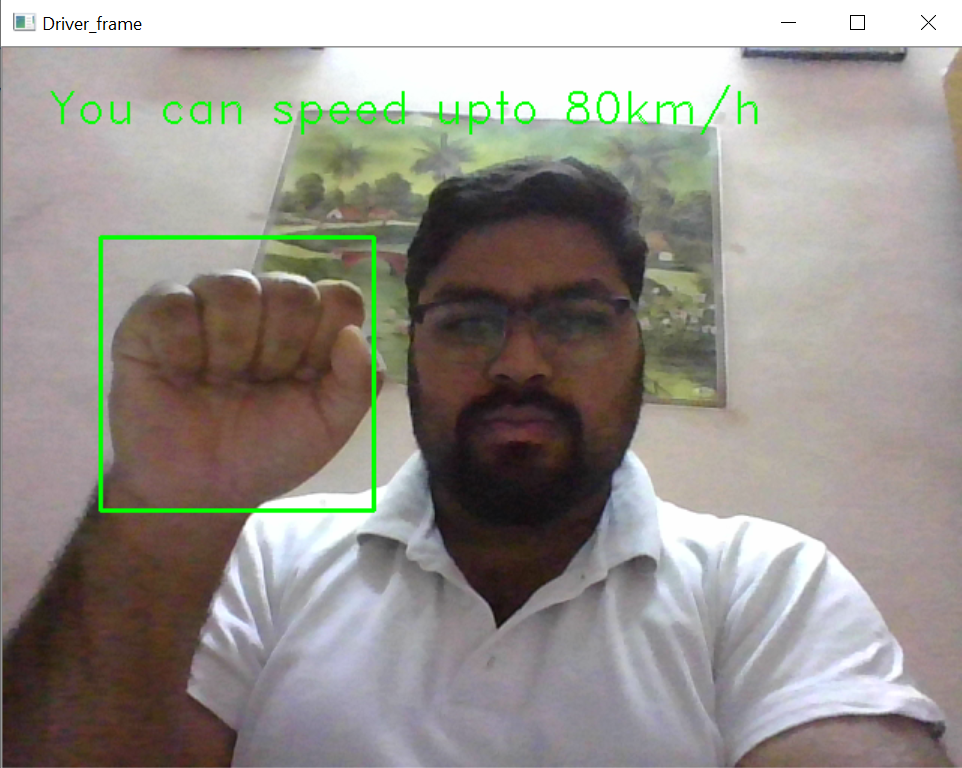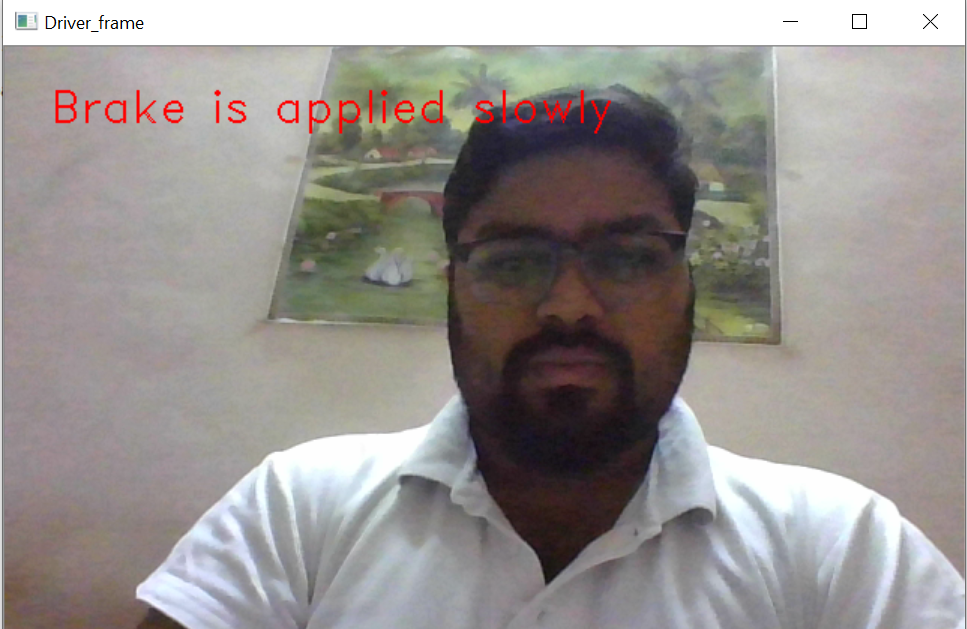# Car driving using hand detection in Python

• Difficulty Level : Expert
• Last Updated : 11 Dec, 2020

In this project, we are going to demonstrate how one can drive a car by just detecting hand gestures on the steering wheel. Let’s say the requirement is something like this –

• If driver wants to start the car then put both of your hands on the steering wheel.
• If someone having no hands on a steering wheel that means brakes of car will be applied slowly.
• If one hand is detected on the steering wheel that means he/she can drive the car upto a certain limit due to safety purpose.
• If someone having both of the hands on the steering wheel that means that he/she can drive at any speed because according to our system you are safe and can safely handle car with both of your hands.

For this project we need to import two Python libraries that is OpenCV and numpy. How to install these two libraries.

```1) pip install opencv-python
2) pip install numpy
```

Below is the implementation :

Code #1:

 `import` `cv2 ` `import` `numpy as np ` ` `  `cap ``=` `cv2.VideoCapture(``0``) ` `hand_cascade ``=` `cv2.CascadeClassifier(``'hand.xml'``) `

Explanation :
We have imported two libraries named opencv and numpy. Then in the next line we use the function `VideoCapture(0)` of opencv and passed the parameter as 0 because your laptop webcam supports port 0 to use the camera. Now, use the function `CascadeClassifier('hand.xml')` and pass the xml file as parameter. Store the file of hand.xml in the same directory as of Python file.

Code #2 :

 `count ``=` `0` ` `  `while``(``True``): ` `    ``ret, frame ``=` `cap.read() ` `    ``gray ``=` `cv2.cvtColor(frame, cv2.COLOR_BGR2GRAY) ` `    ``hands ``=` `hand_cascade.detectMultiScale(gray, ``1.5``, ``2``) ` `    ``contour ``=` `hands ` `    ``contour ``=` `np.array(contour) ` ` `  `    ``if` `count``=``=``0``: ` ` `  `        ``if` `len``(contour)``=``=``2``: ` `            ``cv2.putText(img``=``frame, text``=``'Your engine started'``, ` `                        ``org``=``(``int``(``100` `/` `2` `-` `20``), ``int``(``100` `/` `2``)), ` `                        ``fontFace``=``cv2.FONT_HERSHEY_DUPLEX,  ` `                        ``fontScale``=``1``, color``=``(``0``, ``255``, ``0``)) ` `                         `  `            ``for` `(x, y, w, h) ``in` `hands: ` `                ``cv2.rectangle(frame, (x, y), (x ``+` `w, y ``+` `h), (``0``, ``255``, ``0``), ``2``) ` `            ``count ``+``=` `1` ` `  `    ``if` `count>``0``: ` ` `  `        ``if` `len``(contour)>``=``2``: ` `            ``cv2.putText(img``=``frame, text``=``'You can take your car on long drive'``, ` `                        ``org``=``(``int``(``100` `/` `2` `-` `20``), ``int``(``100` `/` `2``)), ` `                        ``fontFace``=``cv2.FONT_HERSHEY_DUPLEX,  ` `                        ``fontScale``=``1``, color``=``(``255``, ``0``, ``0``)) ` `                         `  `            ``for` `(x, y, w, h) ``in` `hands: ` `                ``cv2.rectangle(frame, (x, y), (x ``+` `w, y ``+` `h), (``0``, ``255``, ``0``), ``2``) ` `             `  ` `  `        ``elif` `len``(contour)``=``=``1``: ` `            ``cv2.putText(img``=``frame, text``=``'You can speed upto 80km/h'``, ` `                        ``org``=``(``int``(``100` `/` `2` `-` `20``), ``int``(``100` `/` `2``)), ` `                        ``fontFace``=``cv2.FONT_HERSHEY_DUPLEX,  ` `                        ``fontScale``=``1``, color``=``(``0``, ``255``, ``0``)) ` `                         `  `            ``for` `(x, y, w, h) ``in` `hands: ` `                ``cv2.rectangle(frame, (x, y), (x ``+` `w, y ``+` `h), (``0``, ``255``, ``0``), ``2``) ` ` `  `        ``elif` `len``(contour)``=``=``0``: ` `            ``cv2.putText(img``=``frame, text``=``'Brake is applied slowly'``, ` `                        ``org``=``(``int``(``100` `/` `2` `-` `20``), ``int``(``100` `/` `2``)), ` `                        ``fontFace``=``cv2.FONT_HERSHEY_DUPLEX,  ` `                        ``fontScale``=``1``, color``=``(``0``, ``0``, ``255``)) ` ` `  `        ``count ``+``=` `1` ` `  `cv2.imshow(``'Driver_frame'``, frame) ` `k ``=` `cv2.waitKey(``30``) & ``0xff` `if` `k ``=``=` `27``: ` `    ``break`

Output :Explanation :
In this code section, we use the counter that can help us to start the car’s engine and after the car starts we use the counting of contours on a steering wheel.